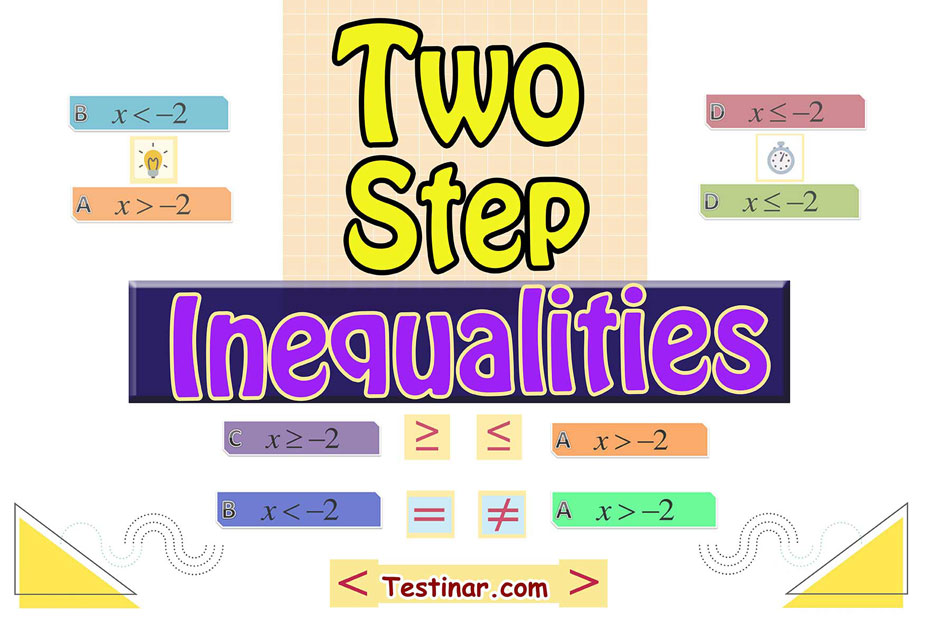## How to Solve Two Step Inequalities?

### Definition of Two Step Inequalities

Two Step Inequalities are inequalities requiring two steps to solve. That means one must add, subtract, multiply, or divide twice to find the answer for the inequality. Whenever one solves each Two Step Inequality they’ll need to either subtract or add first and after that divide or multiply second to find the answer to the inequality.

You’ll see whenever an expression is an inequality via seeing the math symbol in the middle of the expression. If that expression has a greater than, less than, greater than or equal to, or less than or equal to symbol in it, it’s an inequality. If someone multiplies or divides an inequality using a negative number, the inequality sign has to alter its direction.

### Three Simple Steps to solve any kind of Two Step Inequalities Example

1. Subtract or add the constant in order to come up with only the variable on one side of the inequality.
2. Divide or multiply so you can cancel out the coefficient on the term.
3. Should one need to divide or multiply via a negative number, the inequality symbol alters directions.

Example one:
Answer $$3x \ + \ 5 \ < \ 17$$.

Firstly, you must isolate the variable term on one side of the inequality. Then, on the left, $$5$$ gets added to the variable term, $$3x$$. The inverse operation of addition is subtraction. Therefore, you must take $$5$$ from both sides.
$$3x \ + \ 5 \ -5 \ < \ 17 \ -5 \ ⇒ \ 3x \ < \ 12$$
Then, you must take the variable $$x$$ multiplied by $$3$$. The inverse operation of multiplication is division. Therefore, divide both the sides by $$3$$.
$$\frac{3x}{3} \ < \ \frac{12}{3} \ ⇒ \ x \ < \ 4$$
So, the inequality is true for all the values of $$x$$ that are less than $$4$$.
So, the answers to the inequality $$3x \ + \ 5 \ < \ 17$$ are all numbers lower than $$4$$.

Example two:
Answer $$-2x \ - \ 9 \ ≥ \ −7$$.
Firstly, one must isolate the variable term $$-2x$$ which is on the left. The inverse operation of subtraction is addition. Therefore, add $$9$$ to both sides.
$$-2x \ - \ 9 \ + \ 9 \ ≥ \ −7 \ + \ 9 \ ⇒ \ -2x \ ≥ \ 2$$
In order to isolate the variable $$x$$, divide both the sides by $$-2$$.
So, when one multiplies or divides both sides of an inequality using a negative number, you must reverse the inequality.
$$\frac{-2x}{-2} \ ≤ \ \frac{-2}{-2} \ ⇒ \ x \ ≤ \ -1$$
So, the answers to the inequality $$-2x \ - \ 9 \ ≥ \ −7$$ are all numbers less than or equal to $$-1$$.

### Exercises for Two Step Inequalities

1) $$6x + 16 \ \leqslant \ 22$$$$\ \Rightarrow \$$

2) $$4x + 2 \ \leqslant \ 14$$$$\ \Rightarrow \$$

3) $$6x + 9 \ \leqslant \ 45$$$$\ \Rightarrow \$$

4) $$-5x + 14 \ \lt \ -1$$$$\ \Rightarrow \$$

5) $$-4x + 5 \ \geqslant \ -15$$$$\ \Rightarrow \$$

6) $$-5x + 1 \ \gt \ -4$$$$\ \Rightarrow \$$

7) $$-5x + 3 \ \leqslant \ -22$$$$\ \Rightarrow \$$

8) $$-9x + 10 \ \leqslant \ -35$$$$\ \Rightarrow \$$

9) $$-8x + 17 \ \leqslant \ 9$$$$\ \Rightarrow \$$

10) $$-9x + 16 \ \gt \ -2$$$$\ \Rightarrow \$$

1) $$6x + 16 \ \leqslant \ 22$$$$\ \Rightarrow \ \color{red}{6x \ \leqslant \ 22 \ - \ 16}$$$$\ \Rightarrow \ \color{red}{x \ \leqslant \ \frac{6}{6}}$$$$\ \Rightarrow \ \color{red}{x \ \leqslant \ 1}$$
2) $$4x + 2 \ \leqslant \ 14$$$$\ \Rightarrow \ \color{red}{4x \ \leqslant \ 14 \ - \ 2}$$$$\ \Rightarrow \ \color{red}{x \ \leqslant \ \frac{12}{4}}$$$$\ \Rightarrow \ \color{red}{x \ \leqslant \ 3}$$
3) $$6x + 9 \ \leqslant \ 45$$$$\ \Rightarrow \ \color{red}{6x \ \leqslant \ 45 \ - \ 9}$$$$\ \Rightarrow \ \color{red}{x \ \leqslant \ \frac{36}{6}}$$$$\ \Rightarrow \ \color{red}{x \ \leqslant \ 6}$$
4) $$-5x + 14 \ \lt \ -1$$$$\ \Rightarrow \ \color{red}{-5x \ \lt \ -1 \ - \ 14}$$$$\ \Rightarrow \ \color{red}{x \ \gt \ \frac{-15}{-5}}$$$$\ \Rightarrow \ \color{red}{x \ \gt \ 3}$$
5) $$-4x + 5 \ \geqslant \ -15$$$$\ \Rightarrow \ \color{red}{-4x \ \geqslant \ -15 \ - \ 5}$$$$\ \Rightarrow \ \color{red}{x \ \leqslant \ \frac{-20}{-4}}$$$$\ \Rightarrow \ \color{red}{x \ \leqslant \ 5}$$
6) $$-5x + 1 \ \gt \ -4$$$$\ \Rightarrow \ \color{red}{-5x \ \gt \ -4 \ - \ 1}$$$$\ \Rightarrow \ \color{red}{x \ \lt \ \frac{-5}{-5}}$$$$\ \Rightarrow \ \color{red}{x \ \lt \ 1}$$
7) $$-5x + 3 \ \leqslant \ -22$$$$\ \Rightarrow \ \color{red}{-5x \ \leqslant \ -22 \ - \ 3}$$$$\ \Rightarrow \ \color{red}{x \ \geqslant \ \frac{-25}{-5}}$$$$\ \Rightarrow \ \color{red}{x \ \geqslant \ 5}$$
8) $$-9x + 10 \ \leqslant \ -35$$$$\ \Rightarrow \ \color{red}{-9x \ \leqslant \ -35 \ - \ 10}$$$$\ \Rightarrow \ \color{red}{x \ \geqslant \ \frac{-45}{-9}}$$$$\ \Rightarrow \ \color{red}{x \ \geqslant \ 5}$$
9) $$-8x + 17 \ \leqslant \ 9$$$$\ \Rightarrow \ \color{red}{-8x \ \leqslant \ 9 \ - \ 17}$$$$\ \Rightarrow \ \color{red}{x \ \geqslant \ \frac{-8}{-8}}$$$$\ \Rightarrow \ \color{red}{x \ \geqslant \ 1}$$
10) $$-9x + 16 \ \gt \ -2$$$$\ \Rightarrow \ \color{red}{-9x \ \gt \ -2 \ - \ 16}$$$$\ \Rightarrow \ \color{red}{x \ \lt \ \frac{-18}{-9}}$$$$\ \Rightarrow \ \color{red}{x \ \lt \ 2}$$

## Two Step Inequalities Practice Quiz

### SSAT Middle Level Math Prep 2019

$17.99$12.99

### Comprehensive Math Workbook for the DAT Test

$17.99$11.99

### The Most Comprehensive SSAT Upper Level Math Preparation Bundle

$76.99$36.99

### TSI Math Full Study Guide

$25.99$13.99